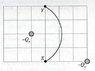# Electric Potential Difference homework help

• cy19861126

## Homework Statement

Two charged rods, each with net charge -Qo, are held in place as shown in the diagram below
a) A small test charge -qo travels from point X toi point Y along the circular arc shown.
i. Draw an arrow at each point (X and Y) to show the direction of the electric force on the test charge at that point.
ii. Is the work done on the charge by the electric field positive, negative, or zero?
iii. Is the electric potential difference positive negative or zero?

b) The test charge is launched from point X with initial speed vo and is observed to pass through point Y. Is the speed of the test charge at point Y greater than, less than or equal to vo?

V = (delta PE)/q
W = Fd

## The Attempt at a Solution

a) i. The electric force at X is pointing at southwest direction and force at Y is pointing at North direction
ii. Positive. W = Fd. For the rod on the left, the force exerted on the object is 0, so the rod has no effect on the test charge. However, the rod on the right exerts a positive force on the test charge. This is because the force direction from the rod and the displacement are pointing at the same direction
iii. Negative. The difference in electric energy is positive because point X is closer to the negative rods than point Y. And since V = (delta PE)/q. Delta PE is positive and q is negative, so the electric potential difference must come out to be negative.

b) Greater than. -PE = KE. If electric potential energy is negative, then KE must be positive. Thus, greater velocity

#### Attachments

•TUT 1.jpg
10.8 KB · Views: 1,486
Last edited:

Hi.Is this a show-case?

Show case? What are you talking about. I put on the questions I have problems on and then I put the answers I thought to be correct. Although I don't expect any of them to be correct.

I edited my question to make it look like a problem instead of a show case

"However, the rod on the right exerts a positive force on the test charge. This is because the force direction from the rod and the displacement are pointing at the same direction"

I think it's negative force, as the charge is negative and negative charge repel negative.

"b) Greater than. -PE = KE. If electric potential energy is negative, then KE must be positive. Thus, greater velocity"

I think is same,the time spent on decelerating the charge is equal to the time spent on accelerating the charge,so end velocity=same.

Others I think assuming those info u gave're correct and based on my limited AS-lv knowledge and stuff are correct.

I think it's negative force, as the charge is negative and negative charge repel negative.
It doesn't matter if you define force to be positive or negative. You can set the coordinate system whichever way you want. But if the direction of force and the displacement traveled are the same, then work is positive. In this case, force and distanced traveled are the same, I think. If I am wrong, can anyone correct it for me?

I think is same,the time spent on decelerating the charge is equal to the time spent on accelerating the charge,so end velocity=same.
I thought that as long as there is constant force, there is always constant acceleration, unless force is changing at some point...

C'mon, can someone answer my question please. I want to understand the problem rather than getting an answer. Any help will be appreciated.